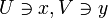Irreducible and Hausdorff implies one-point space

Statement

Suppose$X$ is a non-empty topological space that is both an irreducible space and a Hausdorff space. Then,$X$ must be a one-point space.

Proof

We prove a somewhat modified form: we prove that an Hausdorff space containing two distinct points cannot be irreducible.

Given: A non-empty topological space$X$ that is Hausdorff and has at least two points. Say$x,y$ are two distinct points of$X$.

To prove:$X$ is not irreducible, i.e., it contains two non-empty open subsets with empty intersection.

Proof: Use the definition of Hausdorff to find non-empty open subsets$U \ni x, V \ni y$ that have empty intersection. This completes the proof.